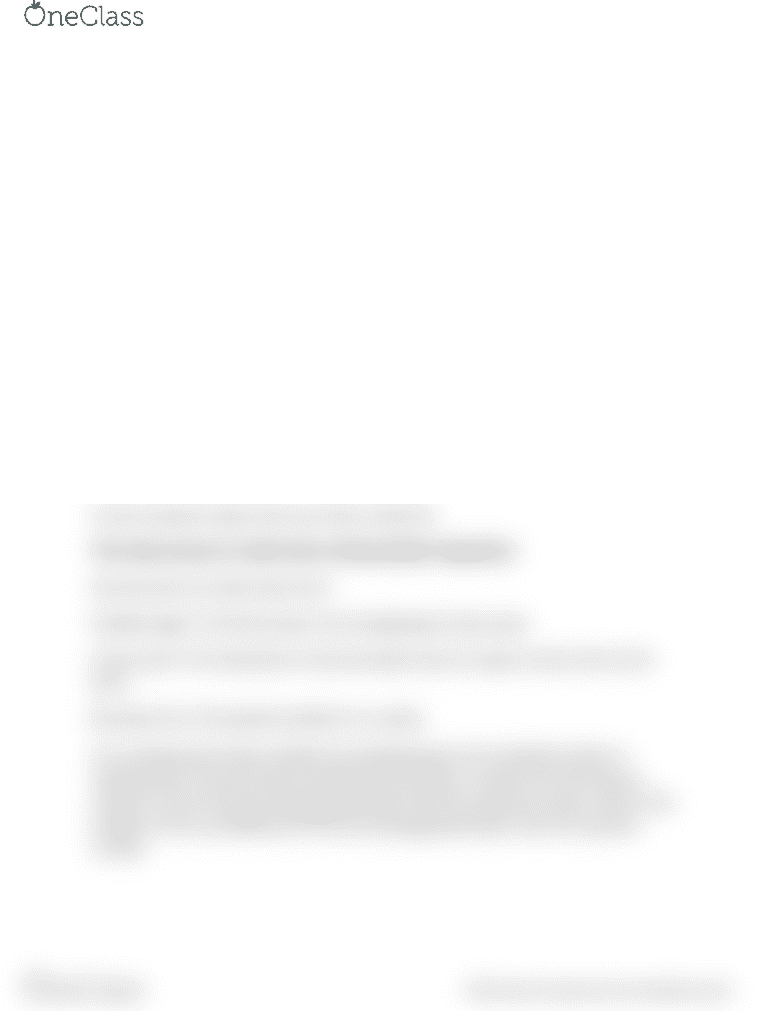# MATH 302 Chapter Notes - Chapter 1.5: Linear Inequality, Free Variables And Bound Variables, Solution Set

27 views1 pages
School
Department
CourseLinear Inequalities
Linear inequality in two variables is simple ax+by <, >, <= or >=, c
You may have a system of linear inequalities,that is you have multiple equations like
these, and you need to find the solution set.
To do this, you look at the answer to each linear inequality and then find the
overlapping answers
Note: If these were not inequalities, but instead equations then if you had two variables,
you may only have been able to solve two equations, or maybe 3 or more, by assuming
one as a free variable.
Anyways, you plot the graph for a linear inequality, this divides the area in two half
planes. Then you look for a test point, on either of the half planes and see if it satisfies
the inequality. That part of the plane is your answer to the inequality.
You do that for all the linear equations, and the overlapping part is your answer.
Also make sure that if the inequality is a simple < or > then you draw a dotted line and if
it has an equals to sign, then your draw a solid line.
This entire process is useful when solving double integrations
Few key terms to make note of are:
Feasible region: The final answer, the overlapping of many areas
Corner point: The intersection of two boundary lines (or I guess more) is the corner
point
Boundary line: The equation plotted on x-y plane
You could go about these problems by breaking down the coordinate system in
individual axis, but that would complicate the problem, as apart from the obvious
solution of one of the axis being greater/lesser than the required number, there is the
problem of the sum/difference of the axis being greater/lesser than the required
number.
find more resources at oneclass.com
find more resources at oneclass.com
Unlock document

This preview shows half of the first page of the document.
Unlock all 1 pages and 3 million more documents.

Already have an account? Log in

# Get access

Grade+
\$10 USD/m
Billed \$120 USD annually
Homework Help
Class Notes
Textbook Notes
40 Verified Answers
Study Guides
1 Booster Class
Class+
\$8 USD/m
Billed \$96 USD annually
Homework Help
Class Notes
Textbook Notes
30 Verified Answers
Study Guides
1 Booster Class